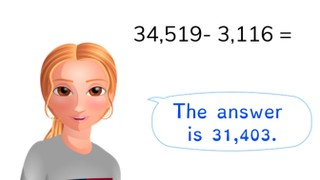Subtracting to 100,000

# Subtracting to 100,000

Students learn to subtract to 100,000.8,000 schools use Gynzy92,000 teachers use Gynzy1,600,000 students use Gynzy

## General

The students learn to subtract to 100,000.

-4.NBT.B.4

## Learning objective

Students will be able to subtract to 100,000 with and without regrouping of the ten-thousands, thousands, hundreds, or tens number.

## Introduction

Have the students solve the story problem.

## Instruction

Show the problem 34,650 - 3,116. Explain that you first subtract the thousands numbers, then the hundreds numbers, tens numbers and ones values. You start to the right of the number line, at 34,650. There, 3,000 is first subtracted, then, you come to 31,650. Next you take away 100, then 10 and finally 6 to reach the answer. After this, show the students the problem 78,748 - 41,627 and have them solve the intermediate steps on their own and fill them in on the lines. Then you show the problem 49,135 - 7,982 and ask the students how they would solve it. Then show the solving strategies used by the children on the whiteboard. Have the students solve the next few problems on their own.

Check whether the students can subtract to 100,000 by asking the following question:
- Which method do you use to solve this problem in steps? (69,485 - 45,122 = ... )

## Quiz

The students are given ten questions in which they first practice solving two problems without regrouping the tens number, hundreds number, thousands number, or ten-thousands number, then a problem with regrouping of the tens number, hundreds number, thousands number and ten-thousands number.

## Closing

Discuss again the importance of being able to subtract to 100,000. As a closing activity have the students spin the spinner and solve the problems.

## Teaching tips

Have students that have difficulty with subtracting to 100,000 first practice subtracting to 10,000.

### The online teaching platform for interactive whiteboards and displays in schools

• Save time building lessons

• Manage the classroom more efficiently

• Increase student engagement